Thermodynamics and Propulsion

# 5.1 Concept and Statements of the Second Law (Why do we need a second law?)

The unrestrained expansion, or the temperature equilibration of the two bricks, are familiar processes. Suppose you are asked whether you have ever seen the reverse of these processes take place? Do two bricks at a medium temperature ever go to a state where one is hot and one is cold? Will the gas in the unrestrained expansion ever spontaneously return to occupying only the left side of the volume? Experience hints that the answer is no. However, both these processes, unfamiliar though they may be, are compatible with the first law. In other words the first law does not prohibit their occurrence. There thus must be some other great principle'' that describes the direction of natural processes, that tells us which first law compatible processes will not be observed. This is contained in the second law. Like the first law, it is a generalization from an enormous amount of observation.

There are several ways in which the second law of thermodynamics can be stated. Listed below are three that are often encountered. As described in class (and as derived in almost every thermodynamics textbook), although the three may not appear to have much connection with each other, they are equivalent.

1. No process is possible whose sole result is the absorption of heat from a reservoir and the conversion of this heat into work. [Kelvin-Planck statement of the second law]2. No process is possible whose sole result is the transfer of heat from a cooler to a hotter body. [Clausius statement of the second law]3. There exists for every system in equilibrium a property called entropy,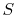, which is a thermodynamic property of a system. For a reversible process, changes in this property are given by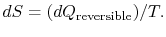The entropy change of any system and its surroundings, considered together, is positive and approaches zero for any process which approaches reversibility.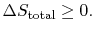For an isolated system, i.e., a system that has no interaction with the surroundings, changes in the system have no effect on the surroundings. In this case, we need to consider the system only, and the first and second laws become: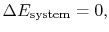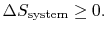For an isolated system the total energy (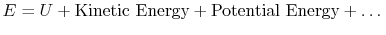) is constant. The entropy can only increase or, in the limit of a reversible process, remain constant.

The limit,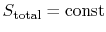or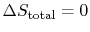, represents the best that can be done. In thermodynamics, propulsion, and power generation systems we often compare performance to this limit to measure how close to ideal a given process is.

All of these statements are equivalent, but 3 gives a direct, quantitative measure of the departure from reversibility.

Entropy is not a familiar concept and it may be helpful to provide some additional rationale for its appearance. If we look at the first law,the term on the left is a function of state, while the two terms on the right are not. For a simple compressible substance, however, we can write the work done in a reversible process as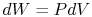, so thatTwo out of the three terms in this equation are expressed in terms of state variables. It seems plausible that we ought to be able to express the third term using state variables as well, but what are the appropriate variables? If so, the termshould perhaps be viewed as analogous towhere the parentheses denote an intensive state variable and the square brackets denote an extensive state variable. The second law tells us that the intensive variable is the temperature,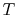, and the extensive state variable is the entropy,. The first law for a simple compressible substance in terms of state variables is thus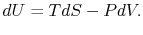(5..1)

Because Eq. 5.1 includes the second law, it is referred to as the combined first and second law. Because it is written in terms of state variables, it is true for all processes, not just reversible ones.

We summarize below some attributes of entropy:

1. Entropy is a function of the state of the system and can be found if any two properties of the system are known, e.g.oror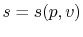.
2.is an extensive variable. The entropy per unit mass, or specific entropy, is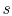.
3. The units of entropy are Joules per degree Kelvin (J/K). The units for specific entropy are J/K-kg.
4. For a system,, where the numerator is the heat given to the system and the denominator is the temperature of the system at the location where the heat is received.
5.for pure work transfer.

Muddy Points

Why is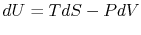always true? (MP 5.1)

What makes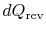different than? (MP 5.2)

UnifiedTP